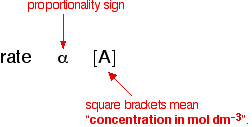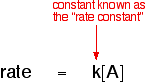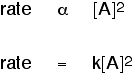# how to determine order of a reaction?tell me in detail.

12 years ago

Orders of reaction are always found by doing experiments. You can't deduce anything about the order of a reaction just by looking at the equation for the reaction.

So let's suppose that you have done some experiments to find out what happens to the rate of a reaction as the concentration of one of the reactants, A, changes. Some of the simple things that you might find are:

One possibility: The rate of reaction is proportional to the concentration of A

That means that if you double the concentration of A, the rate doubles as well. If you increase the concentration of A by a factor of 4, the rate goes up 4 times as well.

You can express this using symbols as:Writing a formula in square brackets is a standard way of showing a concentration measured in moles per cubic decimetre (litre).

You can also write this by getting rid of the proportionality sign and introducing a constant, k.Another possibility: The rate of reaction is proportional to the square of the concentration of A

This means that if you doubled the concentration of A, the rate would go up 4 times (22). If you tripled the concentration of A, the rate would increase 9 times (32). In symbol terms:Generalising this

By doing experiments involving a reaction between A and B, you would find that the rate of the reaction was related to the concentrations of A and B in this way:This is called the rate equation for the reaction.

The concentrations of A and B have to be raised to some power to show how they affect the rate of the reaction. These powers are called the orders of reaction with respect to A and B.

We are all IITians and here to help you in your IIT JEE preparation.

All the best.

If you like this answer please approve it....

win exciting gifts by answering the questions on Discussion Forum

Sagar Singh

B.Tech IIT Delhi